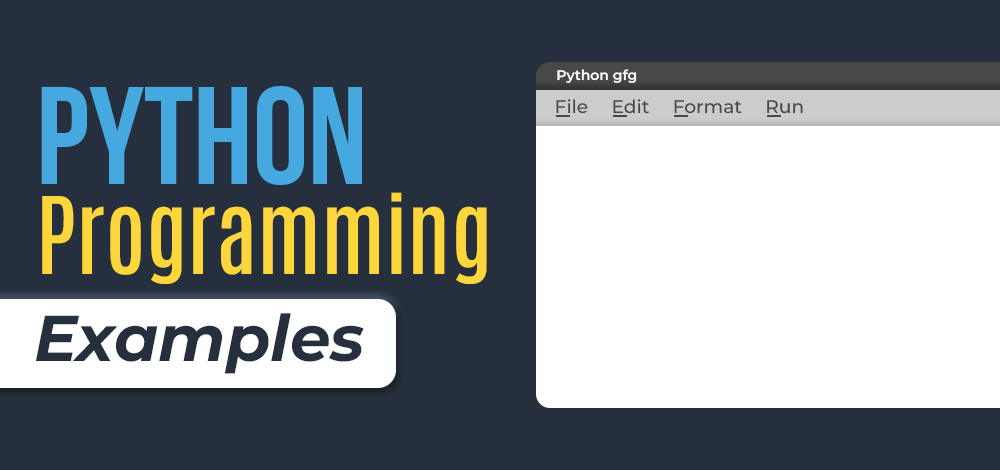# Python Programs

Python Examples: Practice with Python program examples is always a good choice to scale up your logical understanding and programming skills and this article will provide you with the best sets of Python code examples.

The below Python section contains a wide collection of Python programming examples. These Python code examples cover a wide range of basic concepts in the Python language, including List, Strings, Dictionary, Tuple, sets, and many more. Each program example contains multiple approaches to solve the problem.So, without wasting your time start exploring the Python programs and scale up your logical abilities in Python program.

## Basic Programs:

In this section, you will find all the basic Python programming examples. So, explore the section and complete the basic stage of Python programming.

## Array Programs:

To scale up Array logic, try out the below-listed Python array programming examples. Here, you will find all the important Python examples that are related to the Python array concept.

## List Programs:

Explore the below section and try out all the important Python List programming examples.

## Matrix Programs:

Get a detailed list of Python Matrix examples and boost your understanding of matrix concepts in Python.

## String Programs:

If you are looking for Python programming examples that are based on the Python string concept, then scroll down to the below section and practice a wide range of Python string program examples.

## Dictionary Programs:

In this section, you will find out all the important practice sets or examples related to the Python Dictionary concept.

## Tuple Programs:

Explore the wide range of Tuple programs here in this section of Python programming examples.

## Searching and Sorting Programs:

In this section, on searching and sorting examples, we have mentioned all the important example sets of Python searching and sorting to boost your Python programming concept.

## Pattern Printing Programs:

Get a complete list of Python pattern printing examples in the below section.

## Date-Time Programs:

In this section, we have mentioned all important Python program examples that are related to the Python Date-Time concept.

## Python Regex Programs:

To boost Python Regex concept get a list of Python Regex programming examples below.

## Python File Handling Programs:

If you want to scale up your Python file handling concept, explore the below section and find out all the real-life Python programming examples on Python file handling.

## More Python Programs:

Find a more important list of Python programming examples below and upscale your Python programming skills.

If you like GeeksforGeeks and would like to contribute, you can also write an article on https://write.geeksforgeeks.org. See your article appearing on the GeeksforGeeks main page and help other Geeks.